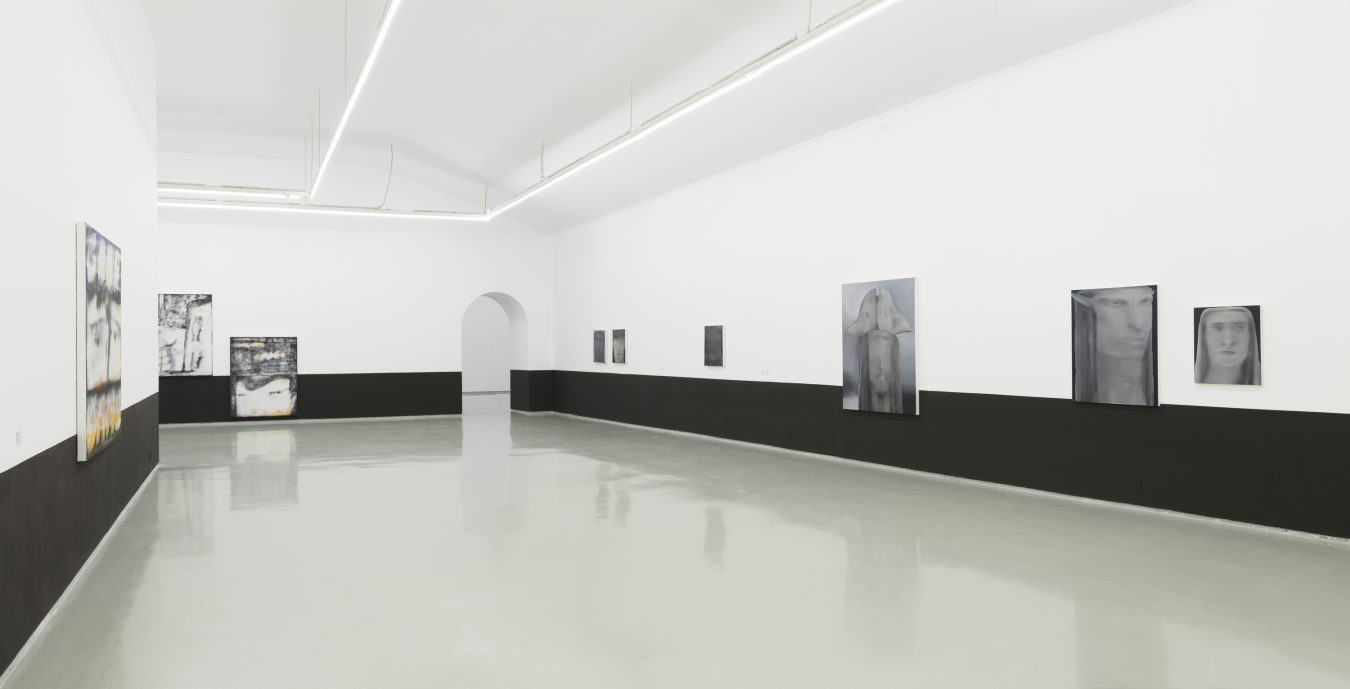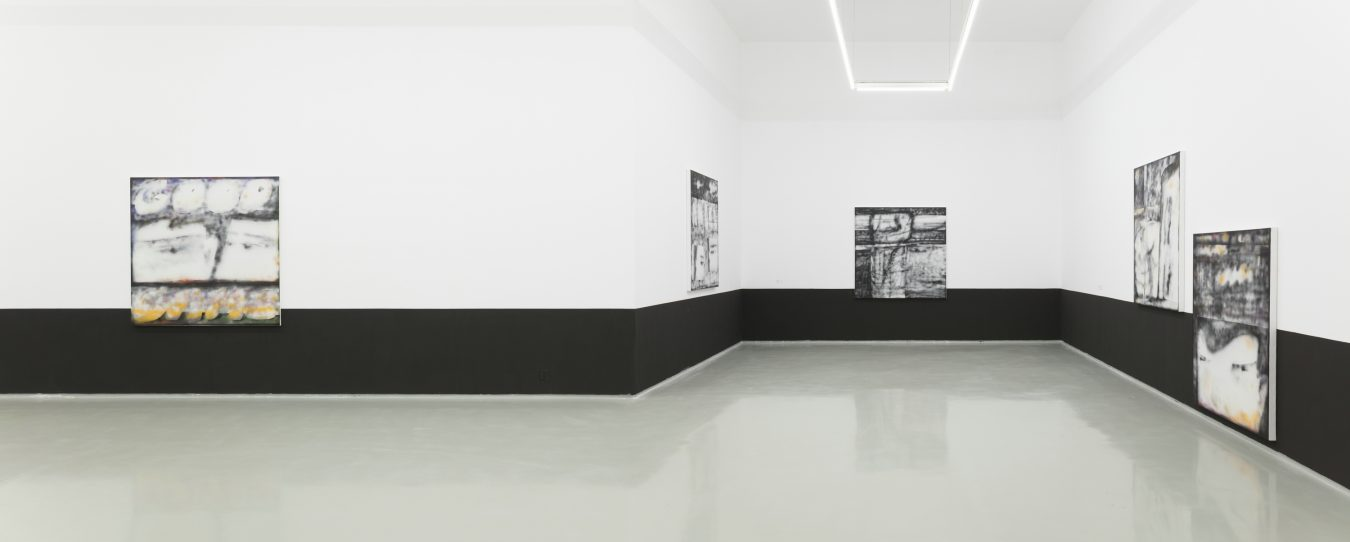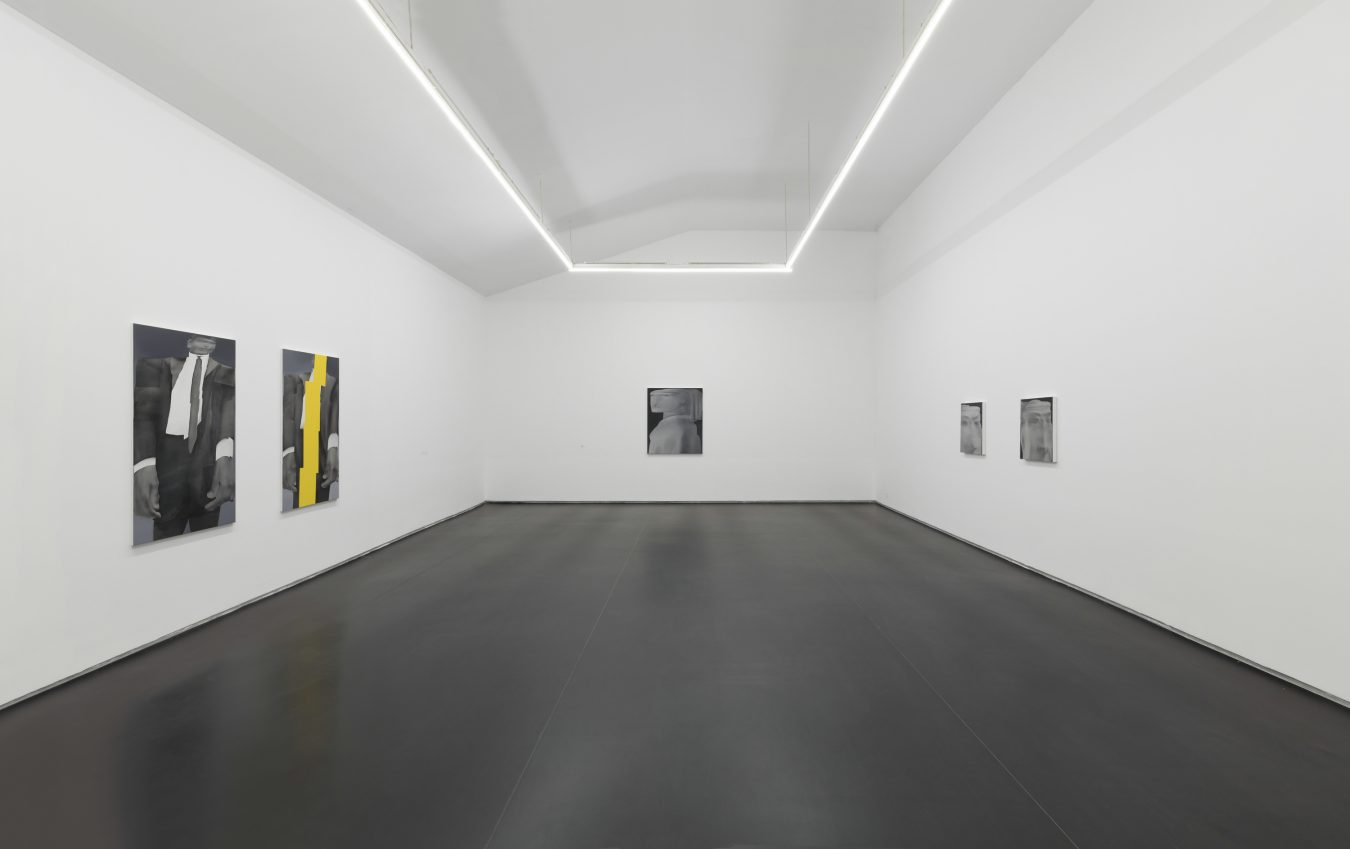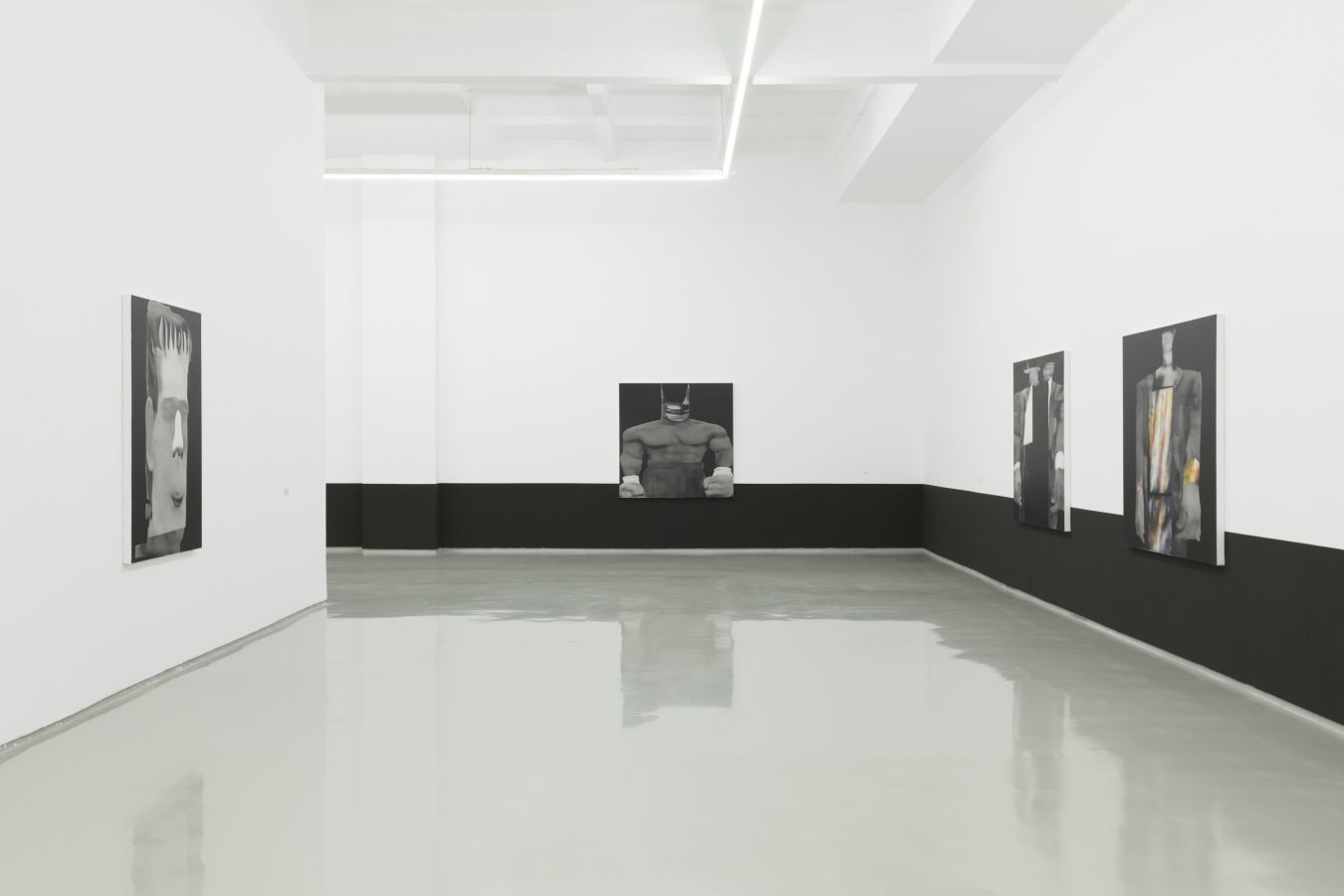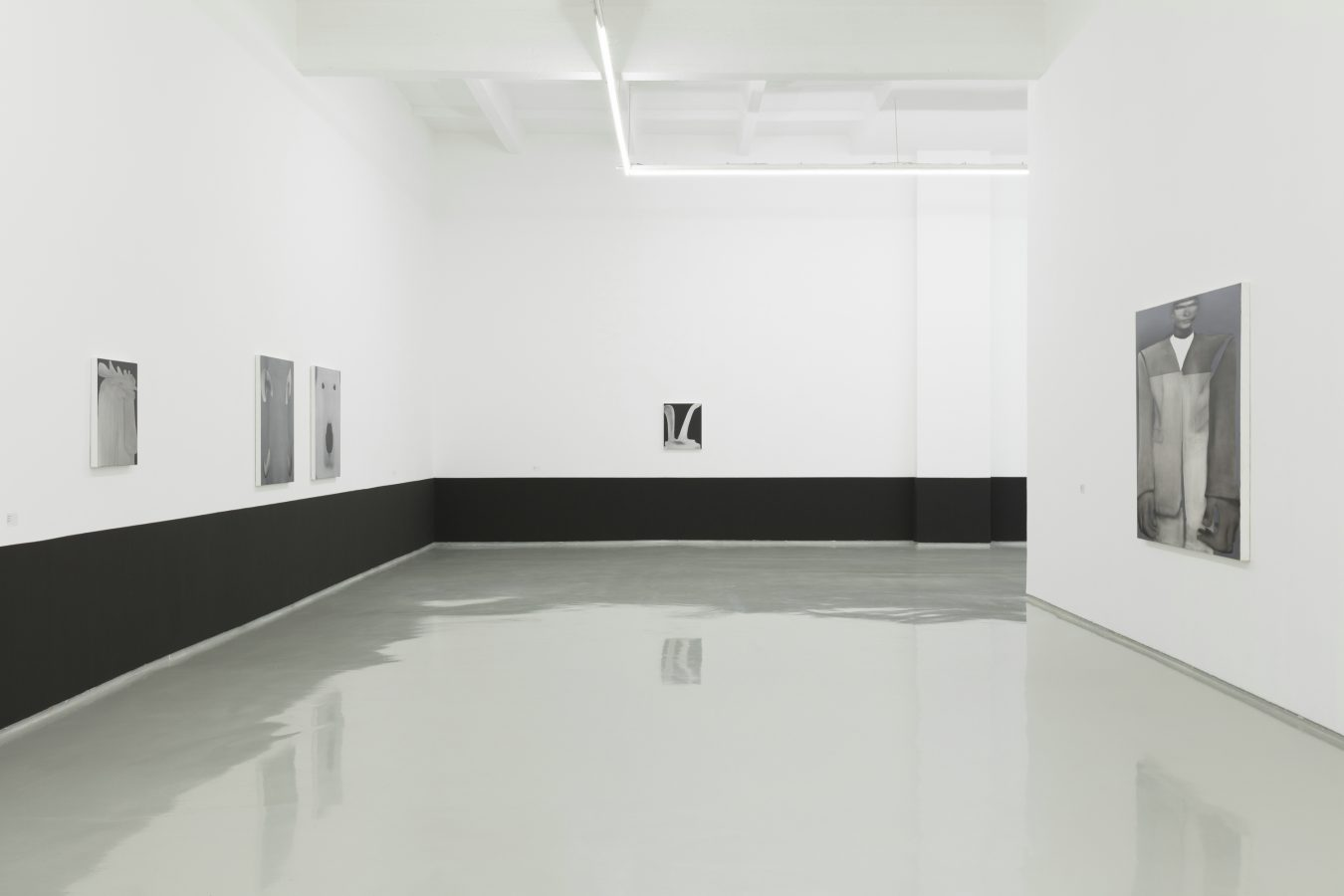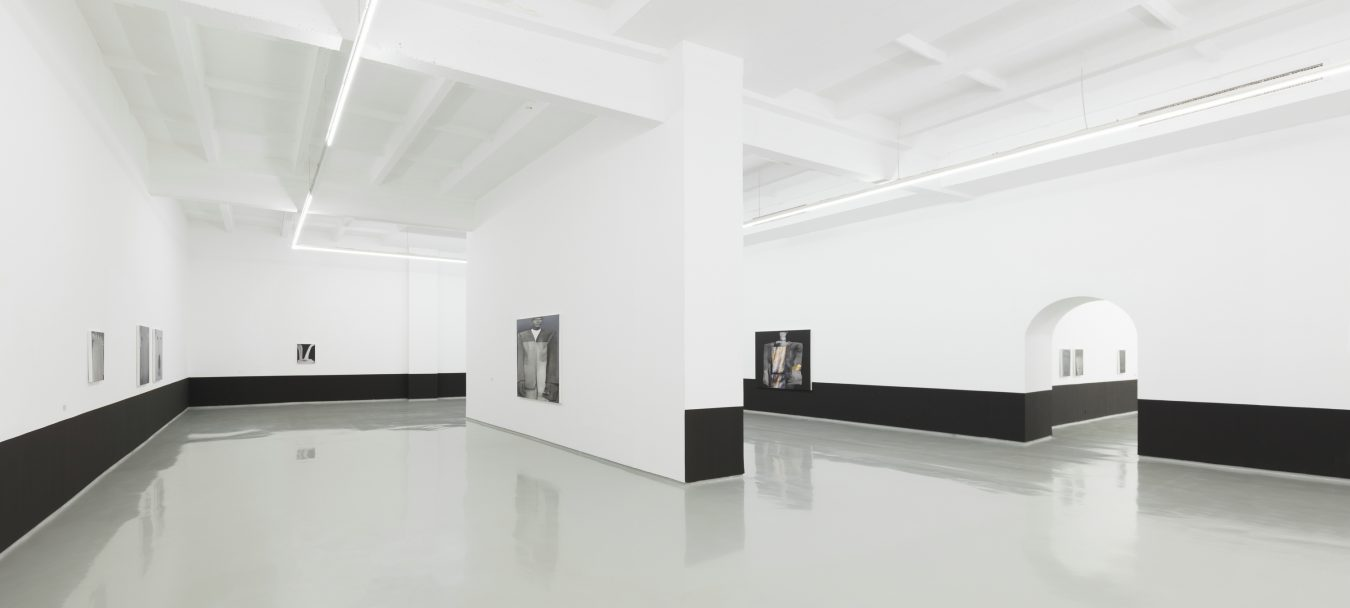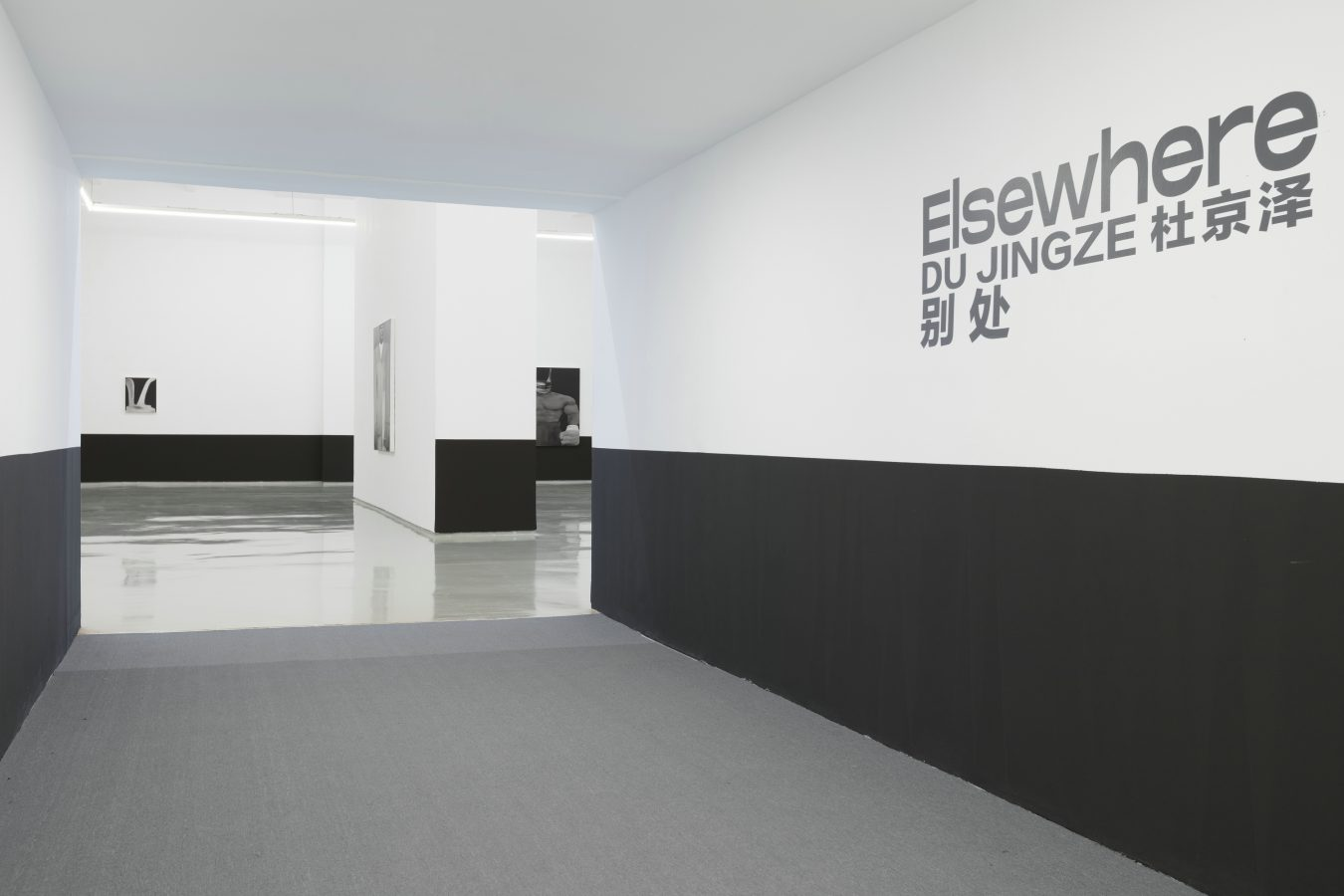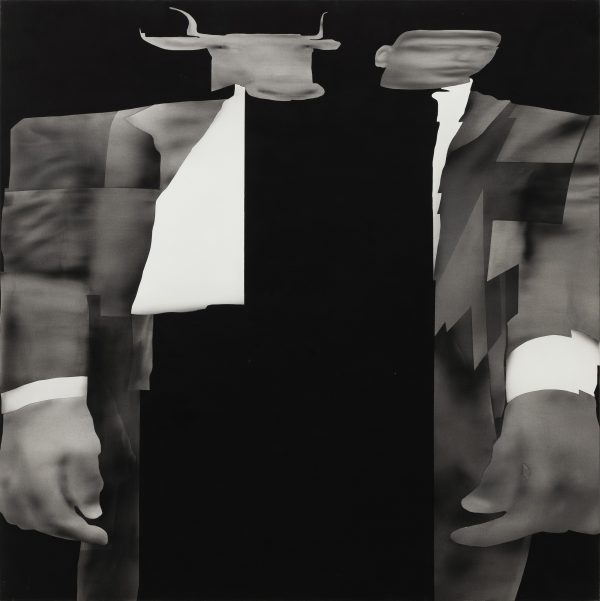2021. 布面油画. 150×150cm.2021. 布面油画. 150×150cm.2021. 布面油画. 120×100cm.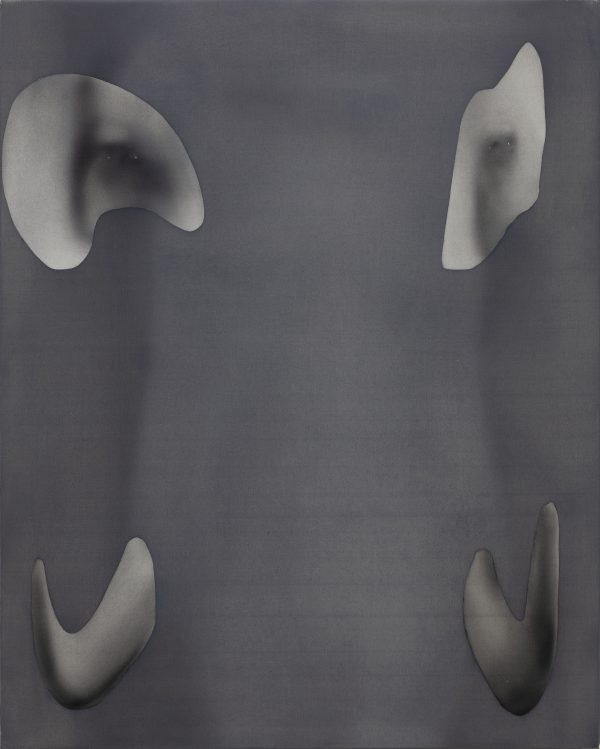2021. 布面油画. 100×80cm.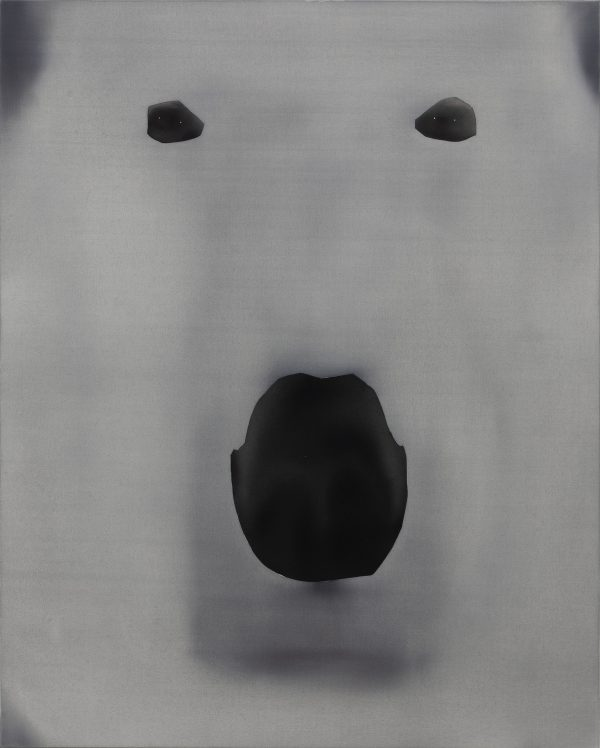2021. 布面油画. 100×80cm.2021. 布面油画. 150×120cm.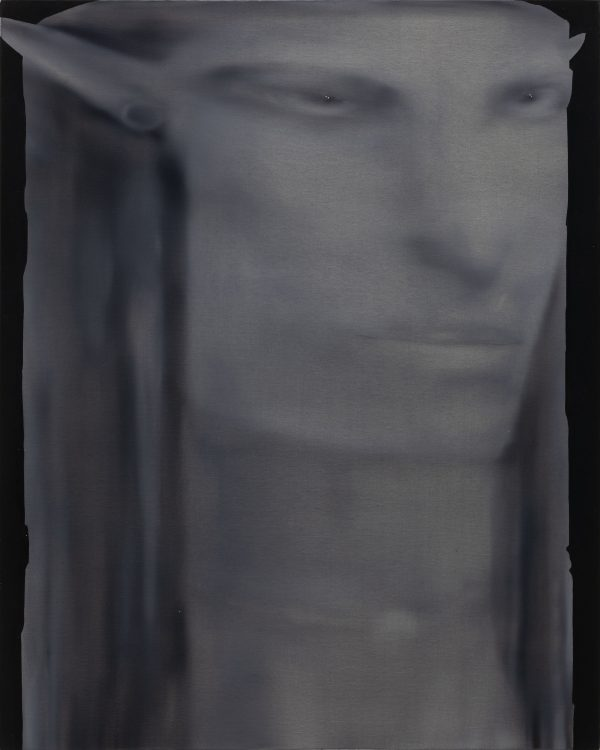2021. 布面油画. 100×80cm.2021. 布面油画. 60×50cm.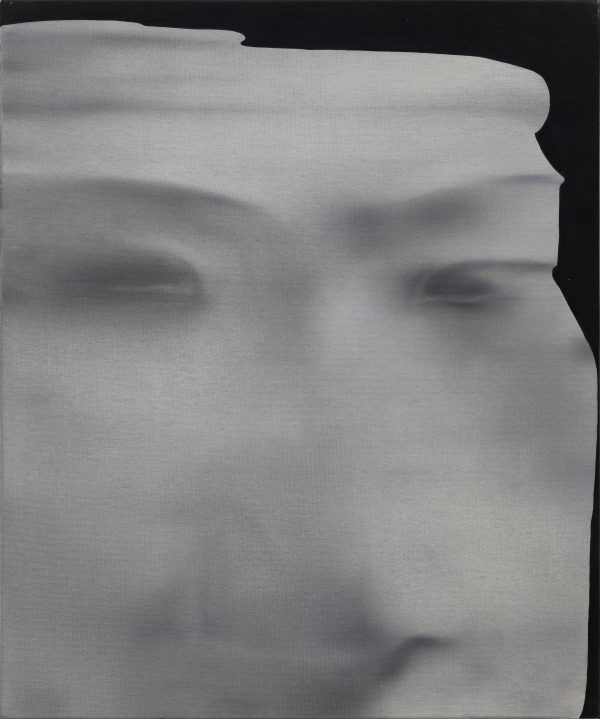2021. 布面油画. 60×50cm.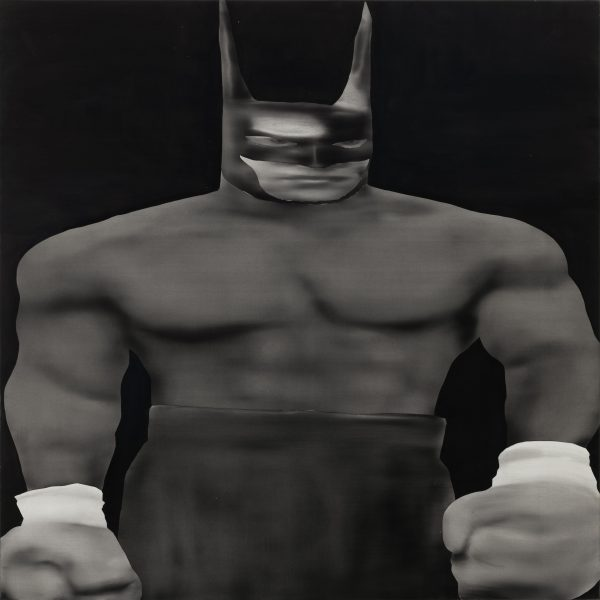2020. 布面油画. 150×150cm.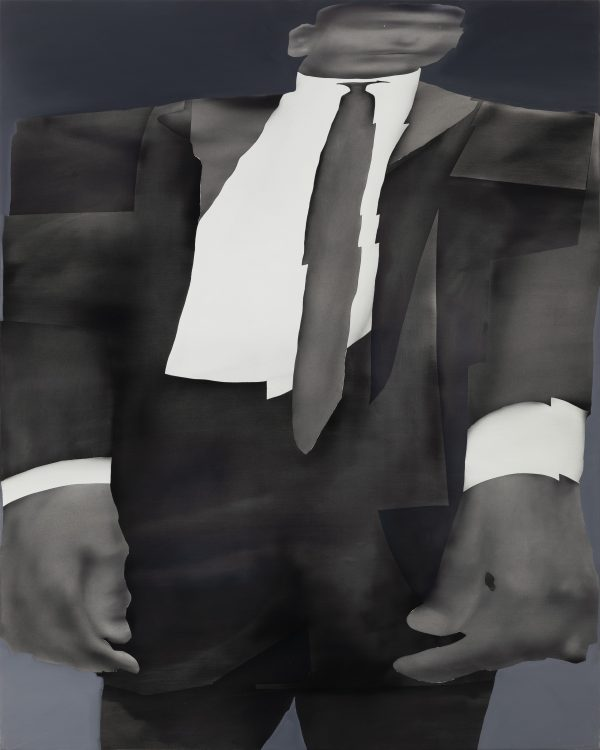2020. 布面油画. 150×120cm.2020. 布面油画. 150×120cm.2020. 布面油画. 150×150cm.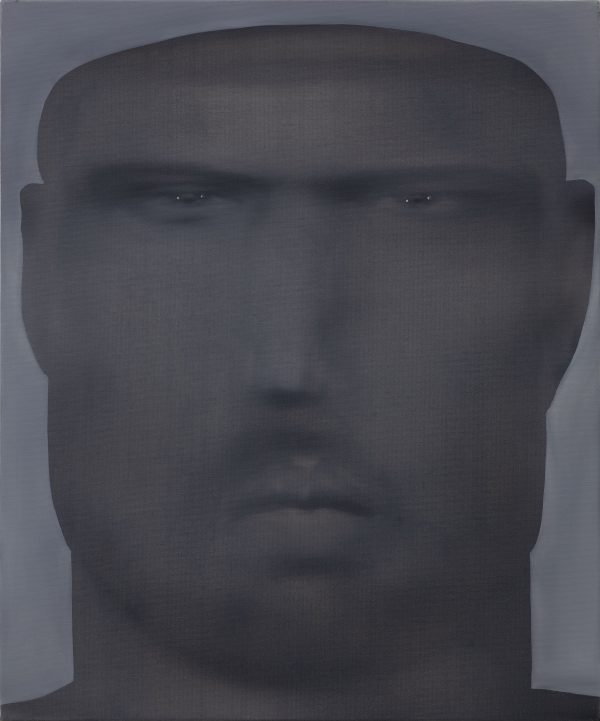2020. 布面油画. 60×50cm.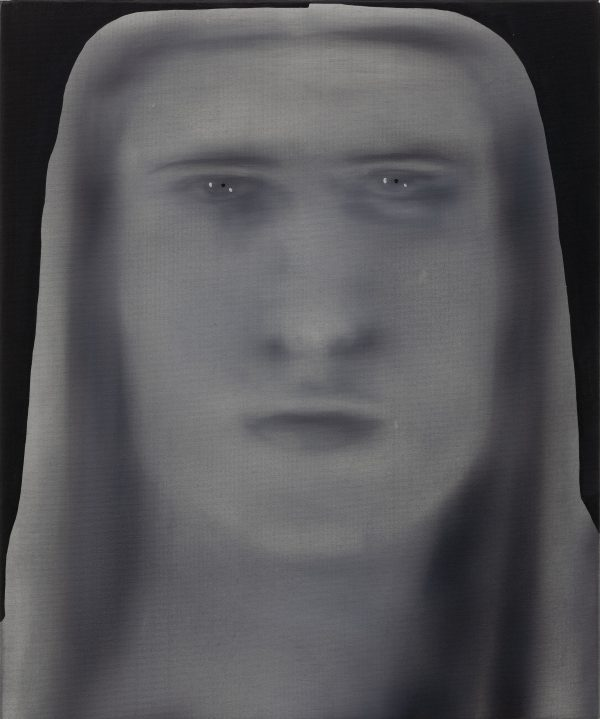2020. 布面油画. 60×50cm.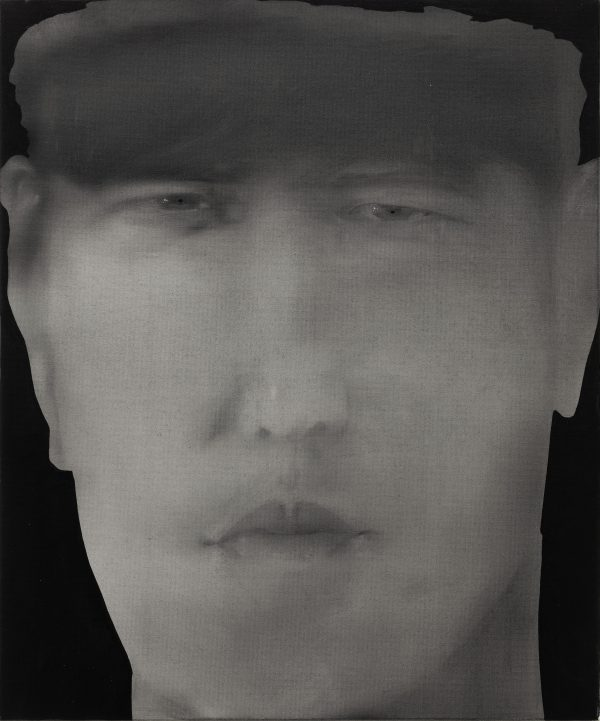2021. 布面油画. 60×50cm.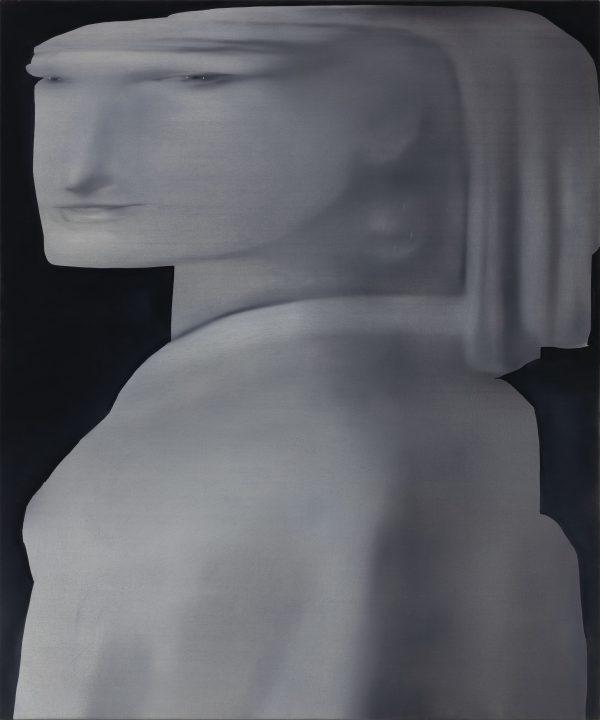2020. 布面油画. 120×100cm.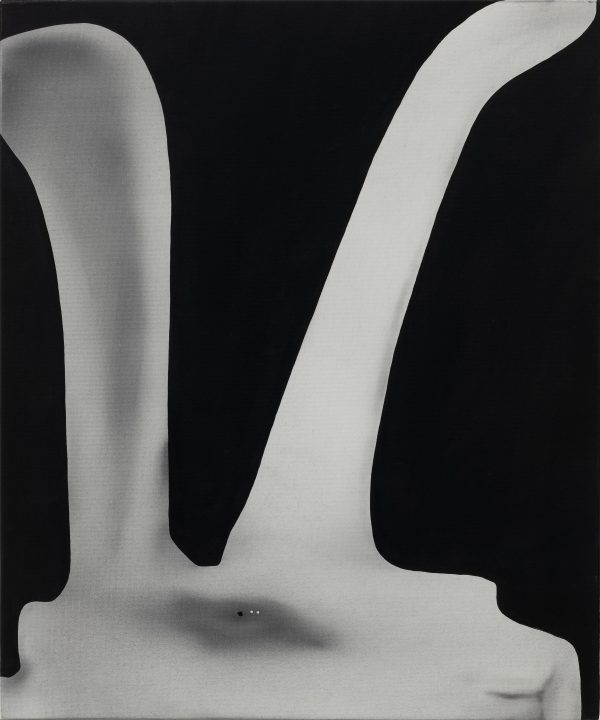2020. 布面油画. 60×50cm.2020. 布面油画. 60×50cm.2020. 布面油画. 60×50cm.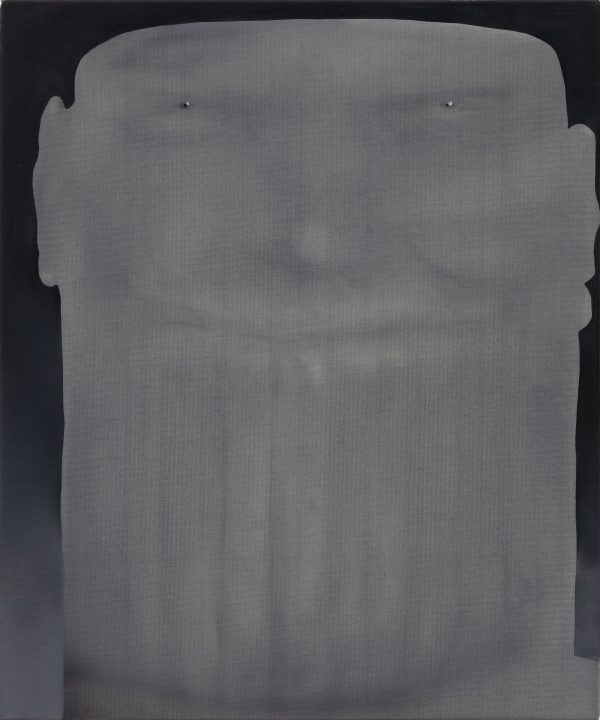2020. 布面油画. 60×50cm.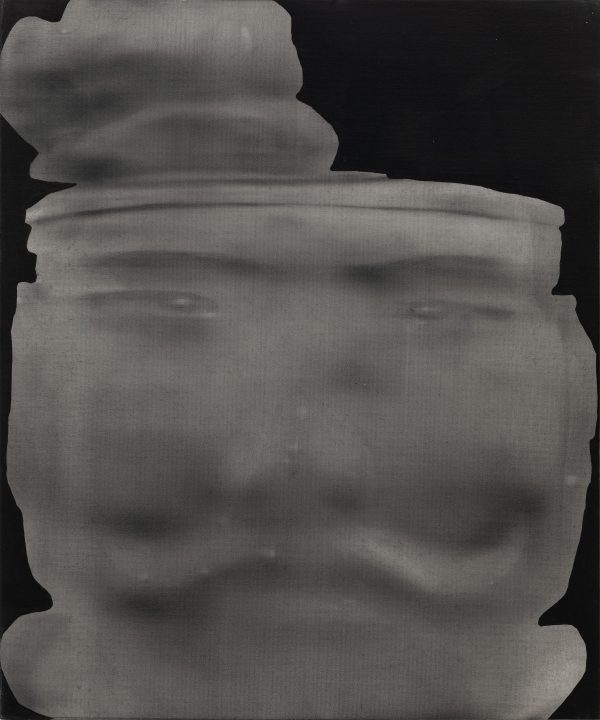Terracotta 1
Du Jingze

2020. Oil on canvas. 60×50cm.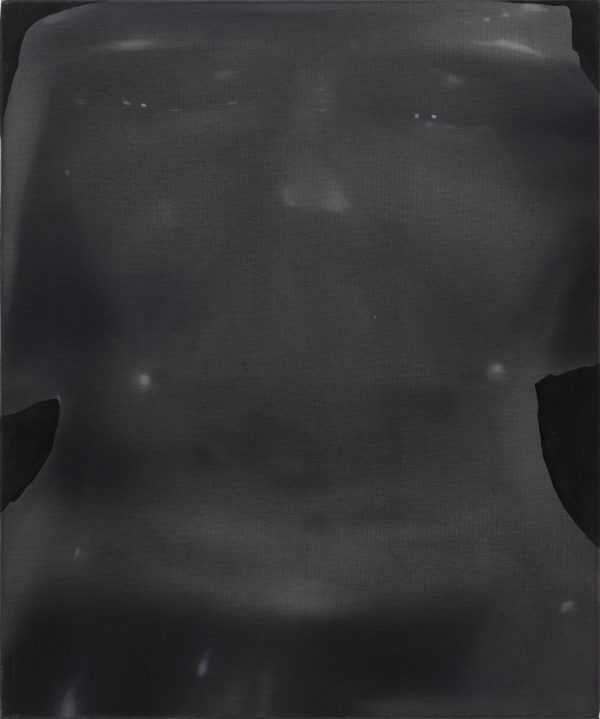2020. 布面油画. 60×50cm.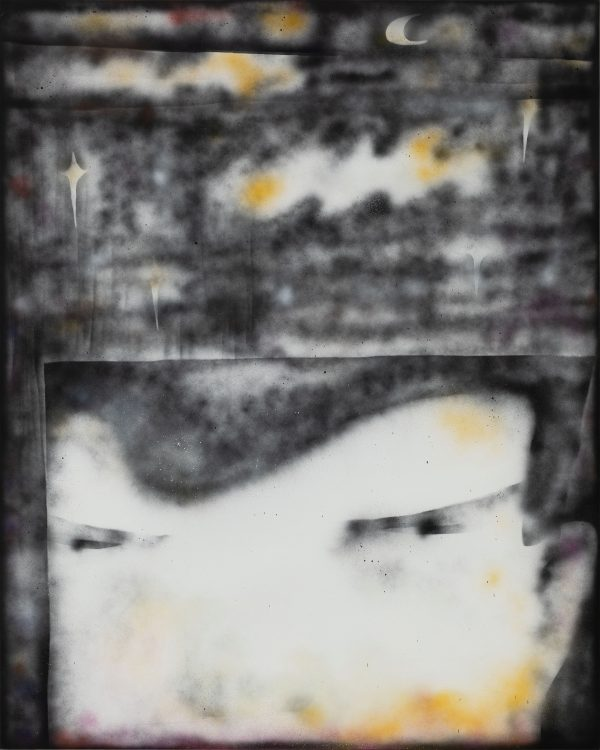To more than inland peace, left by the west wind sweeping overhead

2020. 布面油画. 150×120cm.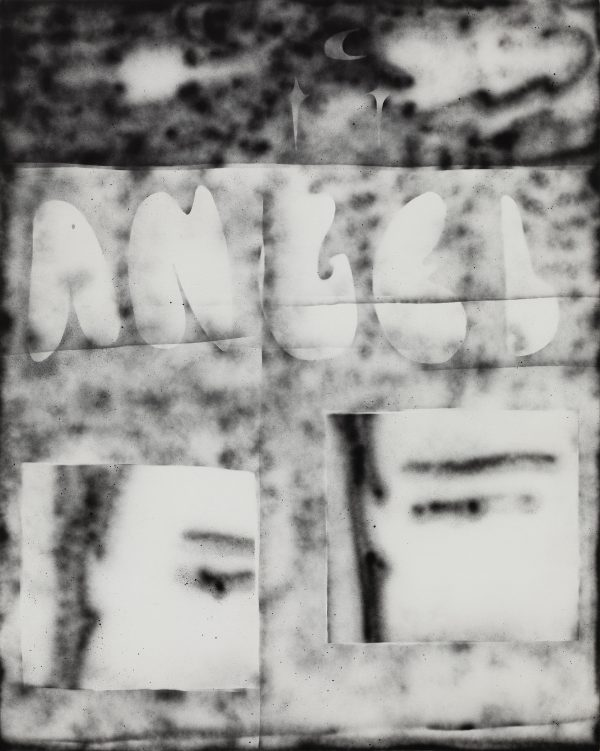2020. 布面油画. 150×120cm.2020. 布面油画. 150×120cm.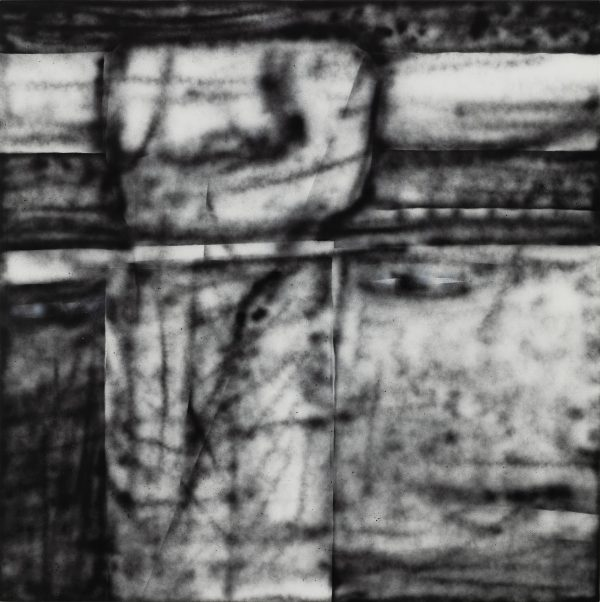2020. 布面油画. 150×120cm.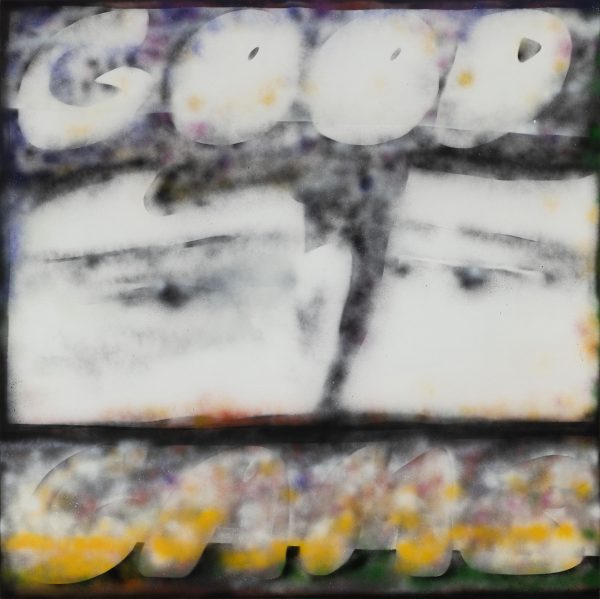GOOD GAME

2020. 布面油画. 150×150cm.